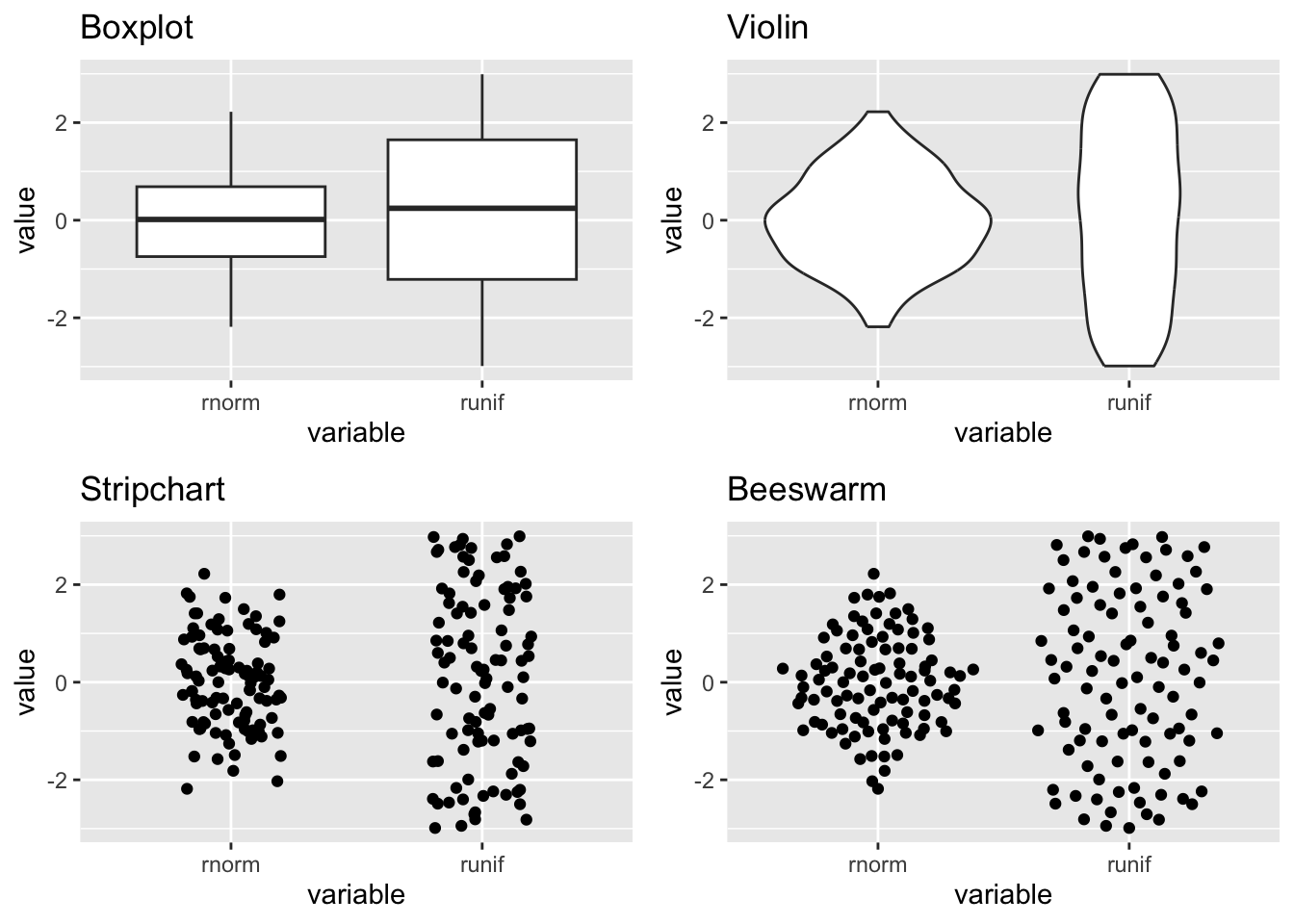# Waggle dance with {ggbeeswarm} and {emoGG}

dataviz
emoGG
emoji
ggbeeswarm
magick
r
Author
Published

November 21, 2018

## tl;dr

Beeswarm plots are a thing. Duncan made a beswarm plot that looks like a beeswarm and I animated it.

## How to plot grouped continuous data?

A boxplot lets you show continuous data split by categories, but it hides the data points and doesn’t tell you much about distribution. A violin chart will show the distribution but you still don’t know about the density of data.

Stripcharts show the data for each category as individual points. The points can be layered on top of each other where they take the same Y value and can be stretched arbitrarily along the X axis.

If you don’t have too much data, or if you sample it, you can stop the data points in a stripchart from overlapping and instead line them up side by side where they take the same Y value. This is called a ‘beeswarm’. Why? Probably because the cloud of data you’re plotting looks a bit like a swarm of bees.

Below is how the plots look side by side.

``````library(ggplot2)     # for plotting
library(ggbeeswarm)  # more on this later
library(cowplot)     # arrange plots

# Create data set
data <- data.frame(
"variable" = rep(c("runif", "rnorm"), each = 100),
"value" = c(runif(100, min = -3, max = 3), rnorm(100))
)

# Generate different plot types
canvas <- ggplot(data, aes(variable, value))
box <- canvas + geom_boxplot() + ggtitle("Boxplot")
violin <- canvas + geom_violin() + ggtitle("Violin")
strip <- canvas + geom_jitter(width = 0.2)  + ggtitle("Stripchart")
bee <- canvas + geom_quasirandom()  + ggtitle("Beeswarm")

# Arrange plots
grid <- plot_grid(box, violin, strip, bee)

print(grid)``````## Obvious next step

We can test this theory by plotting the points as actual bees, lol. Well, emoji bees. Duncan (of {tidyxl} and {unpivotr} fame) did exactly this and tweeted the plot and code.

To summarise, Duncan did this by hacking emojis via {emoGG} into {ggbeeswarm}’s `geom_beeswarm()` function to create `gg_beeswarm_emoji()`. Patent pending, presumably.

## Obvious next next step

Wouldn’t it be great if the little emoji bees moved around a little bit? Almost like a waggle dance?

I cheated a little bit and recoded the `geom_quasirandom()` function from {ggbeeswarm} instead of `geom_beeswarm()`. Why? Beeswarm plots have an inherent ‘neatness’ to them. That is not becoming of a beeswarm. Instead, `geom_quasirandom()` gives you some ‘random’ jitter each time you plot the data.

So we can plot the same data several times and stack the images into a gif. One easy way to do this is via the {magick} package, a re-engineering of the open-source ImageMagick sute of tools from Jeroen Ooms at rOpenSci.

## Code

Attach the packages.

``````library(ggplot2)
library(ggbeeswarm)
library(emoGG)  # remotes::install_github("dill/emoGG")
library(magick)``````

Recode the `geom_quasirandom()` to display emoji, as per Duncan’s tweet.

``````geom_quasi_emoji <- function (
mapping = NULL, data = NULL, width = NULL, varwidth = FALSE,
bandwidth = 0.5, nbins = NULL, method = "quasirandom", groupOnX = NULL,
dodge.width = 0, stat = "identity", position = "quasirandom",
na.rm = FALSE, show.legend = NA, inherit.aes = TRUE, emoji = "1f4l1d", ...
) {

img <- emoji_get(emoji)[]

position <- position_quasirandom(
width = width, varwidth = varwidth,
bandwidth = bandwidth, nbins = nbins, method = method,
groupOnX = groupOnX, dodge.width = dodge.width
)

ggplot2::layer(
data = data, mapping = mapping, stat = stat,
geom = emoGG:::GeomEmoji, position = position, show.legend = show.legend,
inherit.aes = inherit.aes, params = list(na.rm = na.rm, img = img, ...)
)
}``````

It makes sense to use the data that Duncan generated so we can compare the static plot to the animated one.

``````swarm <- data.frame(
"variable" = rep(c("runif", "rnorm"), each = 100),
"value" = c(runif(100, min = -3, max = 3), rnorm(100))
)``````

Let’s define what our plot should look like. `method = "pseudorandom"` is the bit that gives us the jittering.

``````plot <- ggplot(swarm, aes(variable, value)) +
geom_quasi_emoji(emoji = "1f41d", method = "pseudorandom") +
theme(panel.background = element_rect(fill = "skyblue")) +
ggtitle("WAGGLE DANCE")``````

Now we can create a few versions of this plot with different jittering. The plots are magick-class objects made with `image_graph()` from the {magick} package.

We can loop through a few plots, each representing a frame in the final gif.

And now `image_animate()` can be used to combine those magick objects into a gif.

``````waggle_dance <- image_animate(c(t1, t2, t3, t4))
waggle_dance``````

And we can save this with `image_write()`.

``image_write(waggle_dance, "waggle_dance.gif")``

Well done, we got through this without any bee puns.

## Environment

Session info
``Last rendered: 2023-08-05 17:48:04 BST``
``````R version 4.3.1 (2023-06-16)
Platform: aarch64-apple-darwin20 (64-bit)
Running under: macOS Ventura 13.2.1

Matrix products: default
BLAS:   /Library/Frameworks/R.framework/Versions/4.3-arm64/Resources/lib/libRblas.0.dylib
LAPACK: /Library/Frameworks/R.framework/Versions/4.3-arm64/Resources/lib/libRlapack.dylib;  LAPACK version 3.11.0

locale:
 en_US.UTF-8/en_US.UTF-8/en_US.UTF-8/C/en_US.UTF-8/en_US.UTF-8

time zone: Europe/London
tzcode source: internal

attached base packages:
 stats     graphics  grDevices utils     datasets  methods   base

other attached packages:
 cowplot_1.1.1    ggbeeswarm_0.7.2 ggplot2_3.4.2

loaded via a namespace (and not attached):
 vctrs_0.6.3       cli_3.6.1         knitr_1.43.1      rlang_1.1.1
 xfun_0.39         generics_0.1.3    jsonlite_1.8.7    labeling_0.4.2
 glue_1.6.2        colorspace_2.1-0  htmltools_0.5.5   scales_1.2.1
 fansi_1.0.4       rmarkdown_2.23    grid_4.3.1        evaluate_0.21
 munsell_0.5.0     tibble_3.2.1      fastmap_1.1.1     yaml_2.3.7
 lifecycle_1.0.3   vipor_0.4.5       compiler_4.3.1    dplyr_1.1.2
 htmlwidgets_1.6.2 pkgconfig_2.0.3   rstudioapi_0.15.0 beeswarm_0.4.0
 farver_2.1.1      digest_0.6.33     R6_2.5.1          tidyselect_1.2.0
 utf8_1.2.3        pillar_1.9.0      magrittr_2.0.3    withr_2.5.0
 tools_4.3.1       gtable_0.3.3     ``````

CC BY-NC-SA 4.0